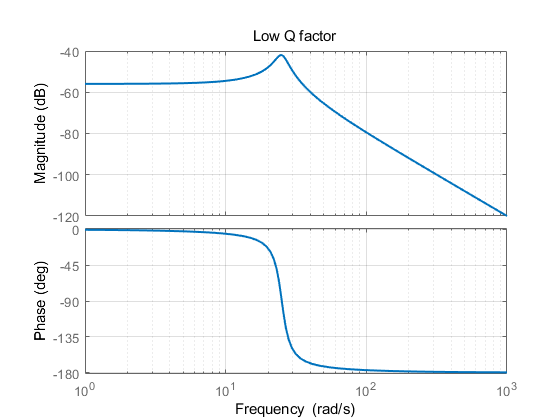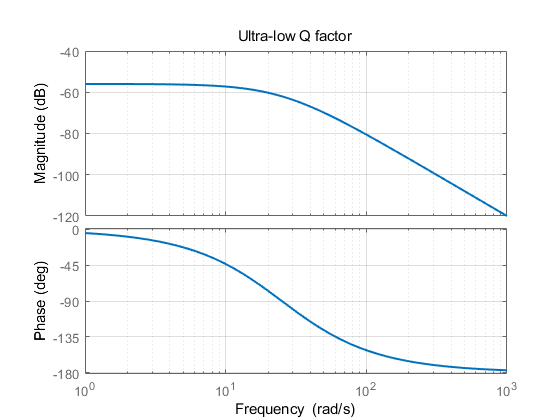# Open-loop excitation resonant gyro phase problem

## From the perspective of control and vibration

Posted by OUC_WYC on September 10, 2021

Tips 若看不到公式，刷新一下即可。

# 控制层面

$G(s)=\frac{1}{s^2+s+628}$

clear;
close all;

num = ;
den = [1,0.1,628];
sys=tf(num,den)                 %输出传递函数
bode(sys)

grid on;
h= findobj(gcf,'type','line');
set(h(3),'linewidth',1.5);      %调整幅值曲线线宽
set(h(4),'linewidth',1.5);      %调整相位曲线线宽
title('High Q factor');


sys =

1
---------------
s^2 + s + 628

Continuous-time transfer function.


# 振动层面

$\ddot{x}+\omega_{0}^{2} x=F \cos \omega t$

$x=A \sin \omega t+B \cos \omega t$

$\left(\omega_{0}^{2}-\omega^{2}\right) A \sin \omega t+\left(\omega_{0}^{2}-\omega^{2}\right) B \cos \omega t=F \cos \omega t$

$%\begin{cases} A=0 \\ B=\frac{F}{\omega_{0}^{2}-\omega^{2}} %\end{cases}$

$x(t)=\frac{F}{\omega_{0}^{2}-\omega^{2}} \cos \omega t$

# 阻尼

$G(s)=\frac{1}{s^2+5s+628}$$G(s)=\frac{1}{s^2+50s+628}$